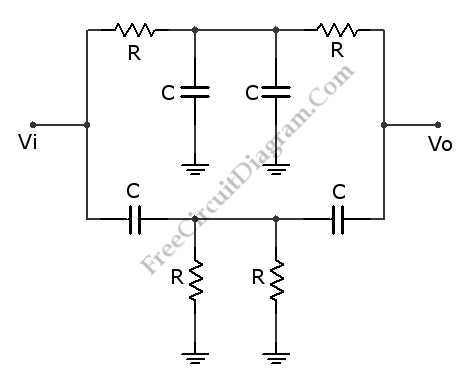# Twin-T Notch FilterThis is a Twin-T Notch Filter circuit. This circuit is a filter that can remove a certain frequency band. This is a passive filter that uses two filters in parallel, high-pass and low-pass. The high-pass filter is lower one and the low-pass filter is the upper one. This filter has a problem with the input resistance. When this filter is used on high frequencies, the input resistance is low about R/4. Beside that, this filter properties depend on the load and the source since this is a passive filter. It can be solved by buffering the filter. Here is the circuit:The minimum gain frequency of this circuit is determined by following equation:
fo = 1 / 2piRC
If the wanted fo is 1591 Hz, the R should be 10K and the C should be 0.01uF. When R=2.7 and C=1uF are used, the filter will block frequencies of 60Hz. By adjusting the frequency until the gain is 0.707 at the low and high points will give the frequencies fL and fH for the -3 dB points. So we can estimate the bandwidth of the filter. The Q of this circuit is determined by following equation: fo/(fH – fL)
And the center frequency divided by the bandwidth.